### OSCR Machine Learning in Python¶

Linear Regression Module

© Kaixin Wang, Fall 2019

Module/Package import

In :
import numpy as np # numpy module for linear algebra
import pandas as pd # pandas module for data manipulation
import matplotlib.pyplot as plt # module for plotting
import seaborn as sns # another module for plotting

In :
import warnings # to handle warning messages
warnings.filterwarnings('ignore')

In :
from sklearn.linear_model import LinearRegression # package for linear model
import statsmodels.api as sm # another package for linear model
import statsmodels.formula.api as smf
import scipy as sp

In :
from sklearn.model_selection import train_test_split # split data into training and testing sets


Dataset import

The dataset that we will be using is the meuse dataset.

As described by the author of the data: "This data set gives locations and topsoil heavy metal concentrations, along with a number of soil and landscape variables at the observation locations, collected in a flood plain of the river Meuse, near the village of Stein (NL). Heavy metal concentrations are from composite samples of an area of approximately 15 m $\times$ 15 m."

In :
soil = pd.read_csv("soil.csv")  # data import
soil.head() # check if read in correctly

Out:
x y cadmium copper lead zinc elev dist om ffreq soil lime landuse dist.m
0 181072 333611 11.7 85 299 1022 7.909 0.001358 13.6 1 1 1 Ah 50
1 181025 333558 8.6 81 277 1141 6.983 0.012224 14.0 1 1 1 Ah 30
2 181165 333537 6.5 68 199 640 7.800 0.103029 13.0 1 1 1 Ah 150
3 181298 333484 2.6 81 116 257 7.655 0.190094 8.0 1 2 0 Ga 270
4 181307 333330 2.8 48 117 269 7.480 0.277090 8.7 1 2 0 Ah 380
In :
soil.shape # rows x columns

Out:
(155, 14)
In :
print(soil.describe())

                   x              y     cadmium      copper        lead  \
count     155.000000     155.000000  155.000000  155.000000  155.000000
mean   180004.600000  331634.935484    3.245806   40.316129  153.361290
std       746.039775    1047.746801    3.523746   23.680436  111.320054
min    178605.000000  329714.000000    0.200000   14.000000   37.000000
25%    179371.000000  330762.000000    0.800000   23.000000   72.500000
50%    179991.000000  331633.000000    2.100000   31.000000  123.000000
75%    180629.500000  332463.000000    3.850000   49.500000  207.000000
max    181390.000000  333611.000000   18.100000  128.000000  654.000000

zinc        elev        dist          om       ffreq  \
count   155.000000  155.000000  155.000000  153.000000  155.000000
mean    469.716129    8.165394    0.240017    7.478431    1.606452
std     367.073788    1.058657    0.197702    3.432966    0.734111
min     113.000000    5.180000    0.000000    1.000000    1.000000
25%     198.000000    7.546000    0.075687    5.300000    1.000000
50%     326.000000    8.180000    0.211843    6.900000    1.000000
75%     674.500000    8.955000    0.364067    9.000000    2.000000
max    1839.000000   10.520000    0.880389   17.000000    3.000000

soil        lime       dist.m
count  155.000000  155.000000   155.000000
mean     1.451613    0.283871   290.322581
std      0.636483    0.452336   226.799927
min      1.000000    0.000000    10.000000
25%      1.000000    0.000000    80.000000
50%      1.000000    0.000000   270.000000
75%      2.000000    1.000000   450.000000
max      3.000000    1.000000  1000.000000

In :
index = pd.isnull(soil).any(axis = 1)
soil = soil[-index]
soil = soil.reset_index(drop = True)

In :
soil.shape

Out:
(152, 14)
In :
n = soil.shape


#### Exploratory Data Analysis (EDA)¶

1. Correlation Heatmap
In :
plt.figure(figsize = (10, 8))
sns.heatmap(soil.corr(), annot = True, square = True, linewidths = 0.1)
plt.ylim(n-1, 0)
plt.xlim(0, n-1)
plt.title("Pearson Correlation Heatmap between variables")
plt.show()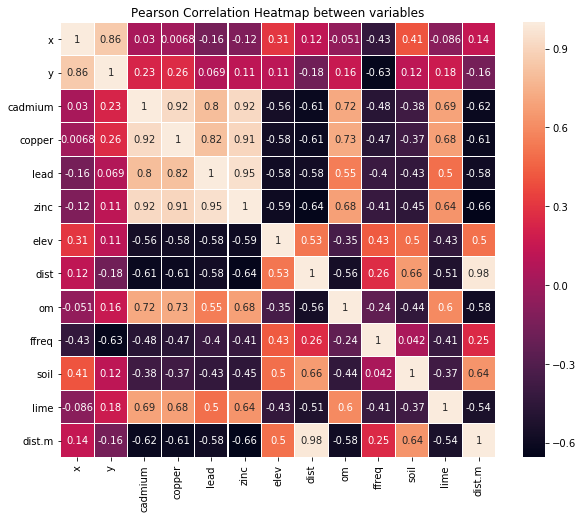In :
plt.figure(figsize = (10, 8))
corr = soil.corr()
mask = np.zeros_like(corr)
mask[np.triu_indices_from(mask)] = True
with sns.axes_style("white"):
sns.heatmap(corr, mask = mask, linewidths = 0.1, vmax = .3, square = True)
plt.ylim(n-1, 0)
plt.xlim(0, n-1)
plt.title("correlation heatmap without symmetric information")
plt.show()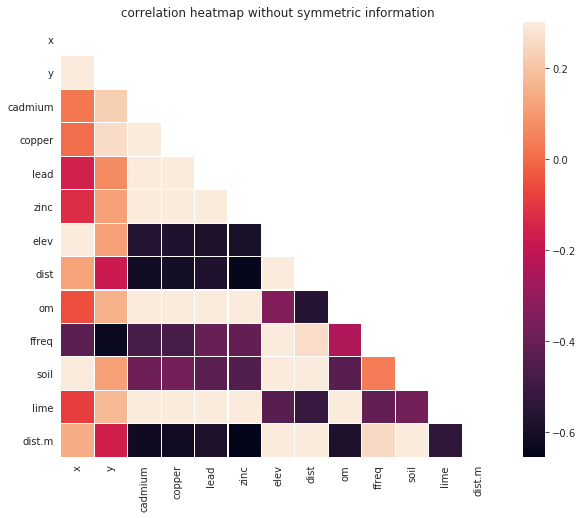In :
fig, axs = plt.subplots(figsize = (8, 12))
plt.subplots_adjust(left=0, bottom=0, right=1, top=1, wspace=0, hspace=0.2)
plt.subplot(2, 1, 1)
# correlation heatmap without annotation
sns.heatmap(soil.corr(), linewidths = 0.1, square = True, cmap = "YlGnBu")
plt.ylim(n-1, 0)
plt.xlim(0, n-1)
plt.title("correlation heatmap without annotation")
plt.subplot(2, 1, 2)
# correlation heatmap with annotation
sns.heatmap(soil.corr(), linewidths = 0.1, square = True, annot = True, cmap = "YlGnBu")
plt.ylim(n-1, 0)
plt.xlim(0, n-1)
plt.title("correlation heatmap with annotation")
plt.show()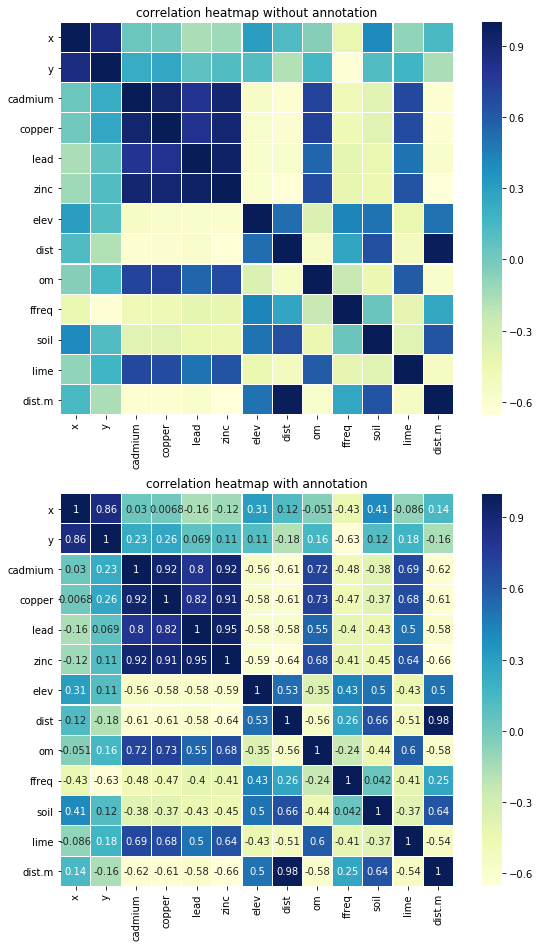2. Boxplots
In :
plt.figure(figsize = (20, 10))
soil.iloc[:, 2:].boxplot()
# soil.iloc[:, 2:].plot(kind = "box")  # 2nd method
plt.title("boxplot for each variable", fontsize = "xx-large")
plt.show()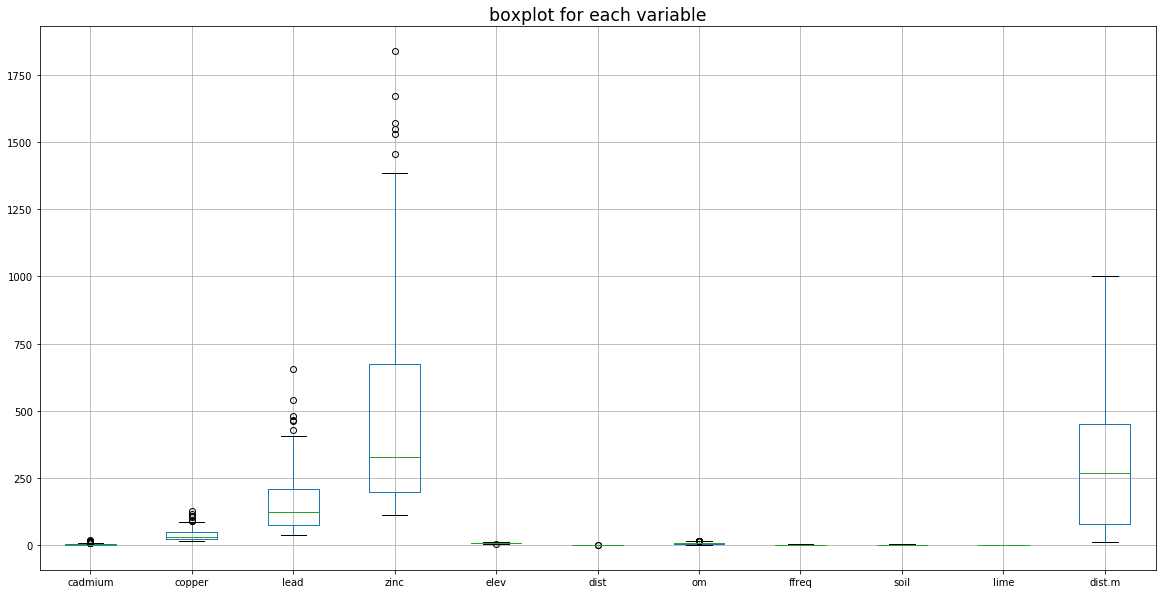In :
fig, axs = plt.subplots(2, int(n/2), figsize = (20, 10))
colors = ['b', 'g', 'r', 'c', 'm', 'y', 'pink']  # to set color

for i, var in enumerate(soil.columns.values):
if var == "landuse":
axs[1, i-int(n/2)].set_xlabel(var, fontsize = 'large')
continue
if i < int(n/2):
axs[0, i].boxplot(soil[var])
axs[0, i].set_xlabel(var, fontsize = 'large')
axs[0, i].scatter(x = np.tile(1, soil.shape), y = soil[var], color = colors[i])
else:
axs[1, i-int(n/2)].boxplot(soil[var])
axs[1, i-int(n/2)].set_xlabel(var, fontsize = 'large')
axs[1, i-int(n/2)].scatter(x = np.tile(1, soil.shape), y = soil[var],
color = colors[i-int(n/2)])

plt.suptitle("boxplot of variables", fontsize = 'xx-large')
plt.show()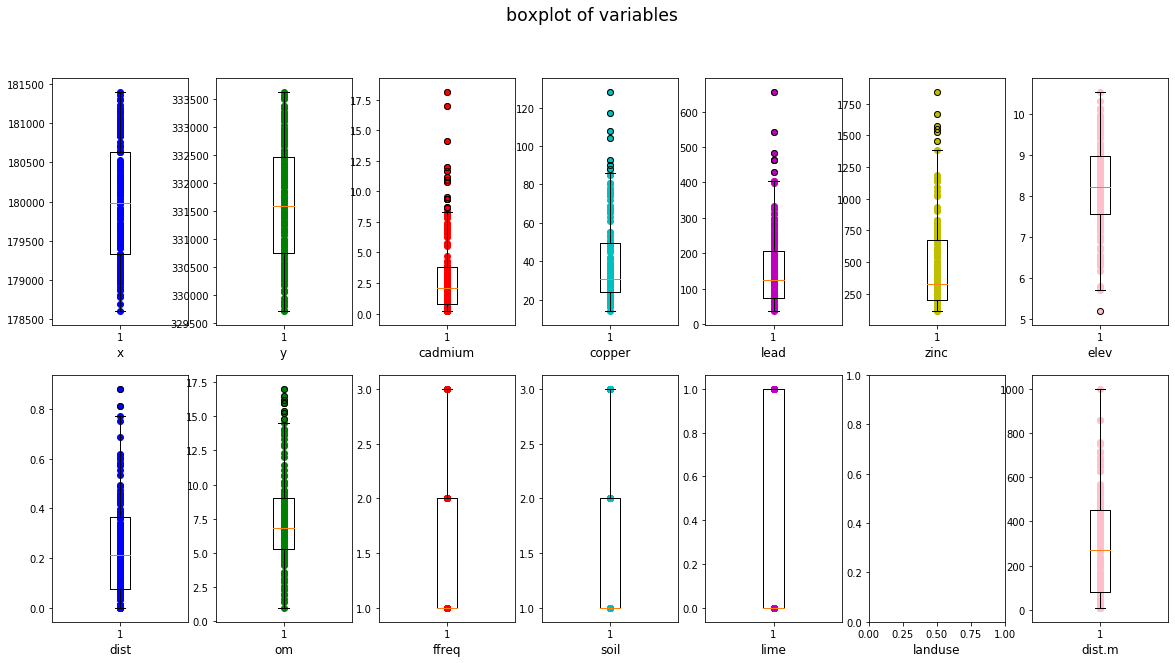3. scatterplots
In :
fig, axs = plt.subplots(2, int(n/2), figsize = (20, 10))
colors = ['b', 'g', 'r', 'c', 'm', 'y', 'k']  # to set color

for i, var in enumerate(soil.columns.values):
if var == "landuse":
axs[1, i-int(n/2)].set_xlabel(var, fontsize = 'large')
continue
if i < int(n/2):
axs[0, i].scatter(soil[var], soil["lead"], color = colors[i])
axs[0, i].set_xlabel(var, fontsize = 'large')
else:
axs[1, i-int(n/2)].scatter(soil[var], soil["lead"], color = colors[i-int(n/2)])
axs[1, i-int(n/2)].set_xlabel(var, fontsize = 'large')

plt.suptitle("scatterplot of lead vs. predictors", fontsize = 'xx-large')
plt.show()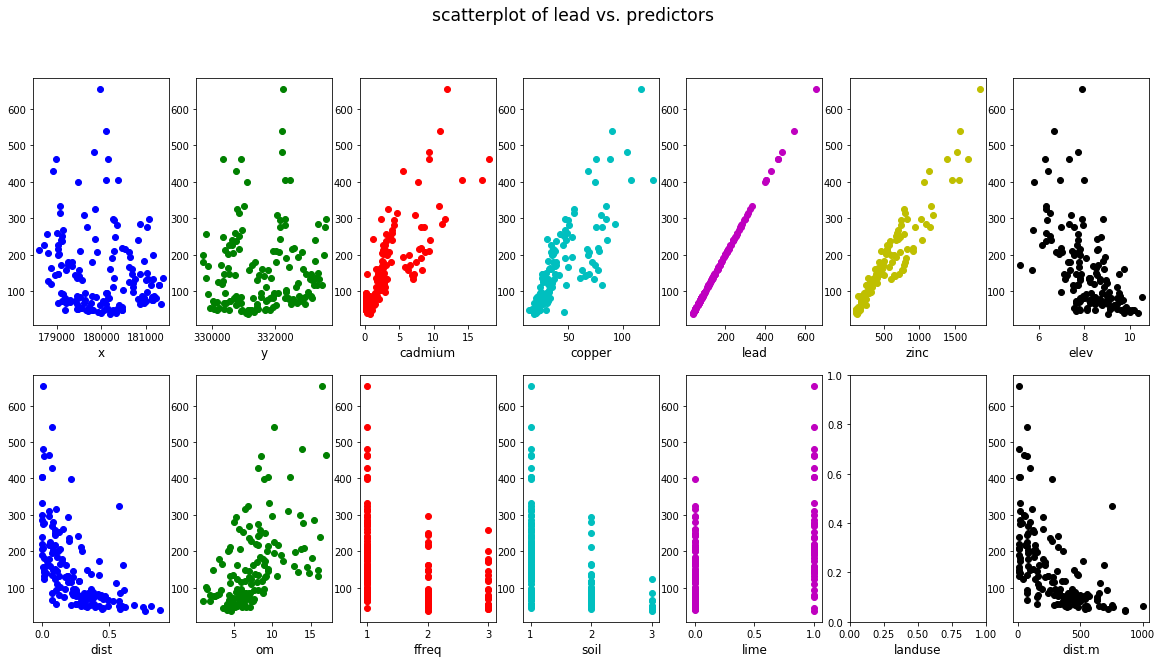4. histograms and density plots
In :
fig, axs = plt.subplots(2, int(n/2), figsize = (20, 10))
colors = ['b', 'g', 'r', 'c', 'm', 'y', 'pink']  # to set color

for i, var in enumerate(soil.columns.values):
if var == "landuse":
axs[1, i-int(n/2)].set_xlabel(var, fontsize = 'large')
continue
if i < int(n/2):
#axs[0, i].hist(soil[var], color = colors[i], alpha = 0.8)
#axs[0, i].set_xlabel(var, fontsize = 'large')
# add density plot
sns.distplot(soil[var], color = colors[i], ax = axs[0, i])
#kde = gaussian_kde(soil[var])
#xaxis = np.linspace(min(soil[var]), max(soil[var]), 20)
#axs[0, i].plot(xaxis, kde(xaxis) * 100)

else:
sns.distplot(soil[var], color = colors[i-int(n/2)], ax = axs[1, i-int(n/2)])

plt.suptitle("histogram and density plot of each variable", fontsize = 'xx-large')
plt.show()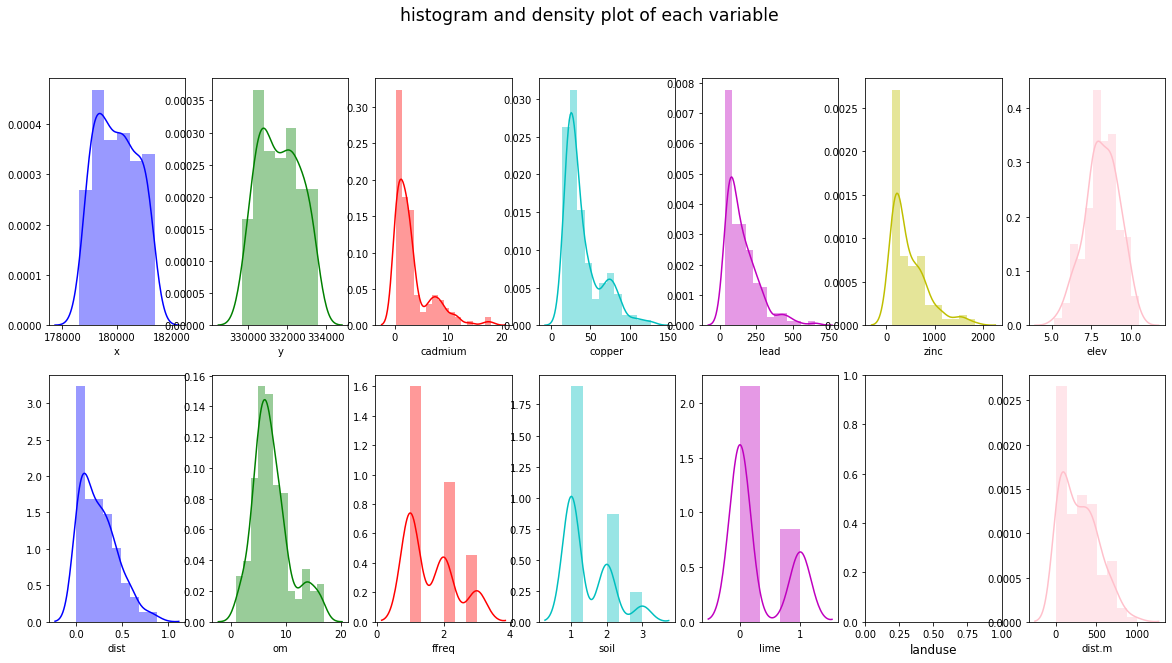Variable Selection and Modeling

Based on the scatterplot of lead vs. predictors:

• cadmium, copper, zinc and om seem to have strong positive correlation with lead
• elev, dist and dist.m seem to have strong negative correlation with lead
• categorical variable lime seems to be a good indicator predictor variable

Therefore, we now build a linear model using 6 predictors: cadmium, copper, zinc, elev, dist and lime.

In :
# variables = ["cadmium", "copper", "zinc", "elev", "dist", "dist.m", "lime"]
variables = ["cadmium", "copper", "zinc", "elev", "dist",  "lime"]

In :
x = soil[variables]
y = soil["lead"]


#### Modeling - building a linear model¶

split the dataset into training and testing set

In :
X_train, X_test, y_train, y_test = train_test_split(x, y, test_size = 0.33, random_state = 42)


build a linear model using sklearn package

In :
model = LinearRegression().fit(X_train, y_train)

In :
# R^2 on training set
R2_train = model.score(X_train, y_train)
R2_train

Out:
0.9483947080189404
In :
# R^2 on testing set
R2_test = model.score(X_test, y_test)
R2_test

Out:
0.964901551899052
In :
# value of intercepts
for var, coef in zip(variables, model.coef_):
print(var, coef)

cadmium -13.391990002100986
copper 0.05365863276389149
zinc 0.42346584485869393
elev -3.2531127698493183
dist 14.509965024252311
lime -23.514058405476963


build a linear model using statsmodel package

In :
df_train = pd.concat([X_train, y_train], axis = 1) # build a dataframe for training set

In :
fullModel = smf.ols("lead ~ cadmium + copper + zinc + elev + lime", data = df_train)

In :
fullModel = fullModel.fit()
print(fullModel.summary())

                            OLS Regression Results
==============================================================================
Dep. Variable:                   lead   R-squared:                       0.948
Model:                            OLS   Adj. R-squared:                  0.945
Method:                 Least Squares   F-statistic:                     346.6
Date:                Sat, 09 Nov 2019   Prob (F-statistic):           2.35e-59
Time:                        21:22:37   Log-Likelihood:                -467.76
No. Observations:                 101   AIC:                             947.5
Df Residuals:                      95   BIC:                             963.2
Df Model:                           5
Covariance Type:            nonrobust
==============================================================================
coef    std err          t      P>|t|      [0.025      0.975]
------------------------------------------------------------------------------
Intercept     23.8739     27.200      0.878      0.382     -30.126      77.874
cadmium      -13.2322      2.240     -5.908      0.000     -17.679      -8.785
copper         0.0522      0.278      0.187      0.852      -0.500       0.605
zinc           0.4188      0.020     20.756      0.000       0.379       0.459
elev          -2.4719      2.895     -0.854      0.395      -8.220       3.276
lime         -24.7610      7.775     -3.185      0.002     -40.196      -9.326
==============================================================================
Omnibus:                        2.077   Durbin-Watson:                   1.947
Prob(Omnibus):                  0.354   Jarque-Bera (JB):                1.494
Skew:                          -0.246   Prob(JB):                        0.474
Kurtosis:                       3.336   Cond. No.                     6.42e+03
==============================================================================

Warnings:
 Standard Errors assume that the covariance matrix of the errors is correctly specified.
 The condition number is large, 6.42e+03. This might indicate that there are
strong multicollinearity or other numerical problems.


diagnostics plots

In :
# diagnosticsPlots function definition
def diagnosticsPlots(predictions, truevalues):
'''
This function will plot the following two diagonistics plots:
1. residuals vs. fitted values plot (plot on the left)
2. qqplot of the residuals (plot on the right)

parameters required:
- predictions: predicted values using the linear regression model
- truevalues:  true values of the response variable

required modules/packages:
- matplotlib.pyplot (plt)
- scipy (sp)

Author: Kaixin Wang
Created: October 2019
'''
residuals = truevalues - predictions
fig, axs = plt.subplots(figsize = (12, 2.5))
plt.subplot(1, 2, 1)
# residuals vs. fitted values
plt.scatter(predictions, residuals)
plt.plot(predictions, np.tile(np.mean(residuals), residuals.shape), "r-")
plt.xlim([np.min(predictions) - 2.5, np.max(predictions) + 2.5])
plt.title("residuals vs. fitted values")
plt.ylabel("residuals")
plt.xlabel("fitted values")
plt.legend(["E[residuals]"])
print("Average of residuals: ", np.mean(residuals))
# qqplot
plot = plt.subplot(1, 2, 2)
sp.stats.probplot(residuals, plot = plot, fit = True)
plt.show()

In :
pred_val = fullModel.fittedvalues.copy()
true_val = y_train.copy()
diagnosticsPlots(pred_val, true_val)

Average of residuals:  -9.60639708594472e-13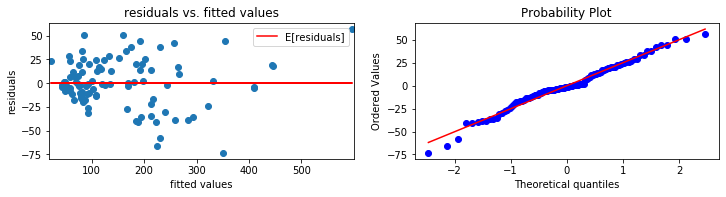training and testing Root-Mean-Square Error (RMSE)

The root-mean-square error of a prediction is calcuated as the following:

$$\text{RMSE} = \frac{\sqrt{\sum_{i=1}^n (y_i - \hat {y_i}) ^ 2}}{n}$$

where $y_i$ is the true value of the response, $\hat {y_i}$ is the fitted value of the response, n is the size of the input

In :
def RMSETable(train_y, train_fitted, train_size, train_R2, test_y, test_fitted, test_size, test_R2):
'''
This function creates a function that returns a dataframe in the following format:
-------------------------------------------------
RMSE     R-squared    size
training set  train_RMSE  train_R2    n_training
testing set   test_RMSE   tesint_R2   n_testing
-------------------------------------------------

parameters required:
- train_y: true values of the response in the training set
- train_fitted: fitted values of the response in the training set
- train_size: size of the training set
- train_R2: R-squared on the training set
- test_y: true values of the response in the testing set
- test_fitted: fitted values of the response in the testing set
- test_size: size of the testing size
- test_R2: R-squared on the testing set

Author: Kaixin Wang
Created: October 2019
'''
train_RMSE = np.sqrt(sum(np.power(train_y - train_fitted, 2))) / train_size
test_RMSE  = np.sqrt(sum(np.power(test_y  - test_fitted, 2))) / test_size
train = [train_RMSE, train_size, train_R2]
test  = [test_RMSE,  test_size,  test_R2]
return pd.DataFrame([train, test], index = ["training set", "testing set"], columns = ["RMSE", "R-squared", "size"])

In :
table1 = RMSETable(y_train.values, model.predict(X_train), R2_train, len(y_train),
y_test.values, model.predict(X_test), R2_test, len(y_test))
print(table1)

                    RMSE  R-squared  size
training set  262.286912   0.948395   101
testing set   159.247070   0.964902    51


#### Model selection¶

Since we are using 5 predictor variables for 152 entries, we can consider using fewer predictors to improve the $R_{adj}^2$, where is a version of $R^2$ that penalizes model complexity.

Selecting fewer predictor will also help with preventing overfitting.

Therefore, observing that zinc, copper and cadmium have relatively stronger correlation with lead, we now build a model using only these three predictors.

using sklearn linear regression module

In :
variables = ["cadmium", "lime", "zinc"]
x = soil[variables]
y = soil["lead"]
X_train, X_test, y_train, y_test = train_test_split(x, y, test_size = 0.33, random_state = 42)

In :
model2 = LinearRegression().fit(X_train, y_train)
# R^2 on training set
R2_train = model2.score(X_train, y_train)
print("Training set R-squared", R2_train)
# R^2 on testing set
R2_test = model2.score(X_test, y_test)
print("Testing set R-squared", R2_test)

Training set R-squared 0.9475953381623881
Testing set R-squared 0.9609072316593821

In :
# value of intercepts
for var, coef in zip(variables, model2.coef_):
print(var, coef)

cadmium -13.093360663968943
lime -23.487105420923648
zinc 0.4236873112817108


using statsmodel ordinary linear regrssion module

In :
df_train = pd.concat([X_train, y_train], axis = 1) # build a dataframe for training set
reducedModel = smf.ols("lead ~ cadmium + C(lime) + zinc", data = df_train)
reducedModel = reducedModel.fit()
print(reducedModel.summary())

                            OLS Regression Results
==============================================================================
Dep. Variable:                   lead   R-squared:                       0.948
Model:                            OLS   Adj. R-squared:                  0.946
Method:                 Least Squares   F-statistic:                     584.7
Date:                Sat, 09 Nov 2019   Prob (F-statistic):           5.98e-62
Time:                        21:22:37   Log-Likelihood:                -468.19
No. Observations:                 101   AIC:                             944.4
Df Residuals:                      97   BIC:                             954.8
Df Model:                           3
Covariance Type:            nonrobust
================================================================================
coef    std err          t      P>|t|      [0.025      0.975]
--------------------------------------------------------------------------------
Intercept        2.6976      4.614      0.585      0.560      -6.461      11.856
C(lime)[T.1]   -23.4871      7.569     -3.103      0.003     -38.509      -8.465
cadmium        -13.0934      1.986     -6.593      0.000     -17.035      -9.152
zinc             0.4237      0.019     22.540      0.000       0.386       0.461
==============================================================================
Omnibus:                        0.749   Durbin-Watson:                   1.968
Prob(Omnibus):                  0.688   Jarque-Bera (JB):                0.395
Skew:                          -0.131   Prob(JB):                        0.821
Kurtosis:                       3.160   Cond. No.                     1.79e+03
==============================================================================

Warnings:
 Standard Errors assume that the covariance matrix of the errors is correctly specified.
 The condition number is large, 1.79e+03. This might indicate that there are
strong multicollinearity or other numerical problems.


diagnostics plots

In :
pred_val = reducedModel.fittedvalues.copy()
true_val = y_train.copy()
diagnosticsPlots(pred_val, true_val)

Average of residuals:  3.392313932510102e-13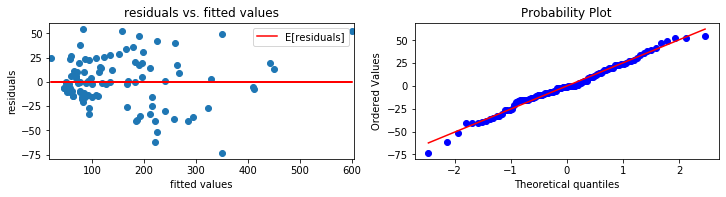In :
table2 = RMSETable(y_train.values, model2.predict(X_train), R2_train, len(y_train),
y_test.values, model2.predict(X_test), R2_test, len(y_test))
print(table2)

                    RMSE  R-squared  size
training set  264.533494   0.947595   101
testing set   168.763005   0.960907    51


#### model evaluation and comparison¶

• model with 5 predictors (full model)
In :
print(table1)

                    RMSE  R-squared  size
training set  262.286912   0.948395   101
testing set   159.247070   0.964902    51

• model with 3 predictors (reduced model)
In :
print(table2)

                    RMSE  R-squared  size
training set  264.533494   0.947595   101
testing set   168.763005   0.960907    51

In :
table = sm.stats.anova_lm(reducedModel, fullModel)
table

Out:
df_resid ssr df_diff ss_diff F Pr(>F)
0 97.0 62835.802512 0.0 NaN NaN NaN
1 95.0 62309.421080 2.0 526.381432 0.401273 0.670596

Comparing the tables obtained above:

• The full model with 6 predictors has lower test RMSE and higher $R^2$ value than the reduced model with 3 predictors
• However, a higher $R^2$ doesn't mean that we favor the full model, since $R^2$ will always increase as the number of predictors used increases. Instead, we will take a look at $R^2_{adj}$, the adjusted $R^2$, which is calculated as
$$R^2_{adj} = 1 - (1 - R^2) \frac{n - 1}{n - p - 1}$$

where $n$ is the sample size and $p$ is the number of predictors used in the model.

• Since $R^2_{adj}$ of the full model is 0.945, while the $R^2_{adj}$ of the reduced model is 0.941, we observe that the model doens't obtain very much improvement by adding three additional predictors.

#### Summary of the module¶

By building two linear regression models using 6 and 3 predictors respectively, we observe that predictor variables cadmium, copper and zinc have very strong positive correlation with the response variable lead. In addition, we also observed that by adding three more predictors, dist, elev and lime, the $R^2_{adj}$ didn't get much improvement comparing to the model with only 3 predictors.

Therefore, the final model that we decided to adopt is the reduced model with 3 predictors:

$$\text{Lead} \sim \text{Cadmium} + \text{lime} + \text{Zinc}$$

Reference:

Dataset: meuse dataset

• P.A. Burrough, R.A. McDonnell, 1998. Principles of Geographical Information Systems. Oxford University Press.
• Stichting voor Bodemkartering (STIBOKA), 1970. Bodemkaart van Nederland : Blad 59 Peer, Blad 60 West en 60 Oost Sittard: schaal 1 : 50 000. Wageningen, STIBOKA.

To access this dataset in R:

install.packages("sp")
library(sp)
data(meuse)

© Kaixin Wang, updated October 2019